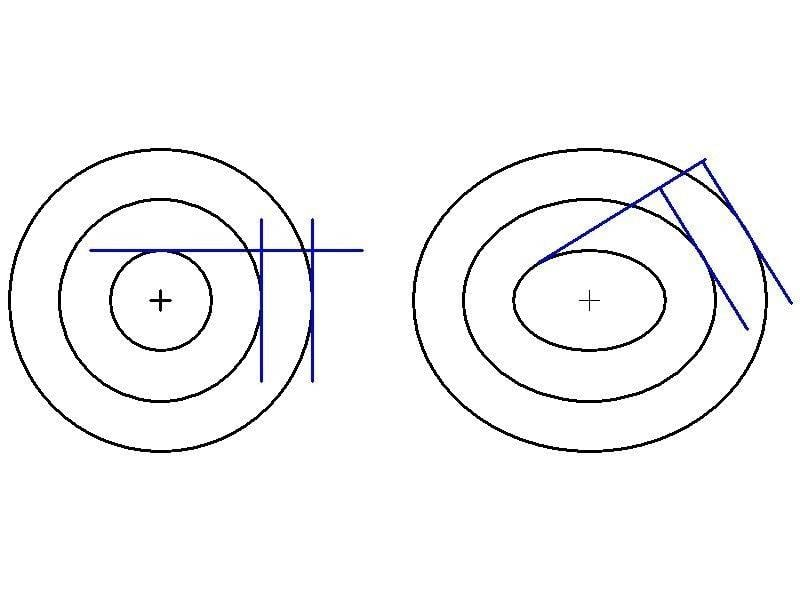# Spiral Equation

IWantToLearn
I am looking for a family of curves where if we consider one curve of them and get the tangent of that curve at any arbitrary point on the curve, then you will always find a point in the other curves where the tangent of this point is perpendicular to the tangent of the first point.

My guess for the solution of this, is that i am looking for a family of spirals that is sink in or out of some point.
but this is just a guess without any rigorous prove,

I tried this:
let ##y_n## be the family of curves, consider two adjacent curves ##y_1 (x)## and ##y_2 (x)##, and that first derivatives (slopes of the tangents) are ##y^\prime_1## and ##y^\prime_2##

for those two tangents to be perpendicular we must have ##y^\prime_1 y^\prime_2 = -1##

lets consider ##S## an equal distance around the curves, where ##S_1 = S_2 = S##, then we have :

##S_1 = \int_{x_1}^{x_2} \sqrt{1+{y^\prime_1}^2} \, dx##
##S_2 = \int_{x_3}^{x_4} \sqrt{1+{y^\prime_2}^2} \, dx##

Assuming we know all the integration boundaries ##x_1,x_2,x_3,x_4##
so we can write

##\int_{x_1}^{x_2} \sqrt{1+{y^\prime_1}^2} \, dx = \int_{x_3}^{x_4} \sqrt{1+\frac{1}{{y^\prime_1}^2}} \, dx##

but i don't know what to do next?

Gold MemberIWantToLearn
thank you for your interest in the question, i was not quite clear in stating the problem, by family of curves i mean a family curves that all intersect at one single point, and they are identical such that you can have them all by rotating one of them around an axis at the point of intersection.

something like this